Compressibility & Consolidation of Soils

# Compressibility & Consolidation of Soils - Notes | Study Soil Mechanics - Civil Engineering (CE)

 Table of contentsCompressibility and Consolidation 51 Crore+ students have signed up on EduRev. Have you?

Compressibility and Consolidation 5

1. Coefficient of Compressibility (av)
av = e- e2- σ1
e1 = Void ratio at effective stress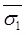e2 =Void ratio at effective stress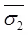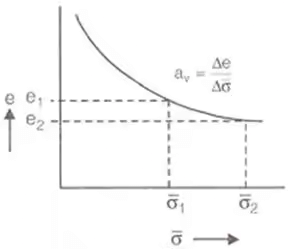ΔV/V0 = ΔH/H0
ΔV = Change in volume in m3, or cm3
V0 = Initial volume in m3 or cm3.
ΔH = Change in depth in 'm' or 'cm'.
H0 = original depth in 'm' or 'cm'.
2. Coefficient of Compression (Cc)
(i)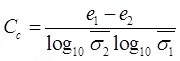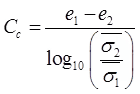(ii) Cc = 0.009(WL-10)
For undisturbed soil of medium sensitivity.
WL = % liquid limit.
(iii) C= 0.009(WL-7)
For remolded soil of low sensitivity
(iv) Cc = 0.40(e0-0.25)
For undisturbed soil of medium sensitivity e0 = Initial void ratio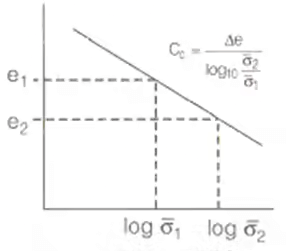(v) For remoulded soil of low sensitivity.
Cc = 1.15(e- 0.35)
(vi) Cc = 0.115w where, w = Water content
3. Over consolidation ratio
O.C.R = Maximum effective stress applied in the past/Existing effective stress
O.C.R > 1 For over consolidated soil.
O.C.R = 1 For normally consolidated soil.
O.C.R < 1 For under consolidated soil.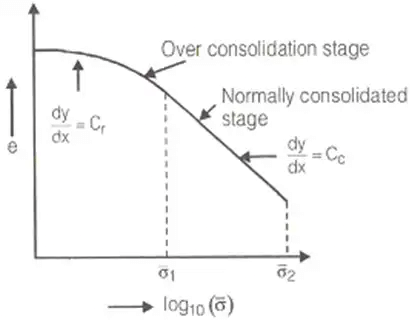Differential Equation of 1-D Consolidation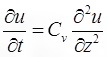where, u = Excess pore pressure.

∂u / ∂t  = Rate of change of pore pressure
Cv = Coefficient of consolidation
∂u / ∂z = Rate of change of pore pressure with depth.

1. Coefficient of volume compressibility mv = av/1+e0 where, e0 = Initial void ratio
mv = Coefficient of volume compressibility
Compression modulus
Ec = 1/mwhere, Ec =Compression modulus.
2. Degree of consolidation
(i) %U = (1-(U/U1)x100) where,
%U = % degree of consolidation.
U = Excess pore pressure at any stage.
U1 =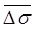= Initial excess pore pressure
at t = 0, u = u1 ⇒ %u = 0%
at t = ∞, u = 0 ⇒ %u = 100%
(ii) %u = (e0-e/e0-ef)x100 where,
ef = Void ratio at 100% consolidation.
i.e. of t = ∞
e = Void ratio at time 't'
e0 = Initial void ratio i.e., at t = 0
(iii) %u = (Δh / ΔH) x 100 where,
ΔH = Final total settlement at the end of completion of primary consolidation i.e.,
at t = ∞
Δh = Settlement occurred at any time 't'.
3. Time factor
Tv = Cv.(t/d2) where, TV = Time factor
CV = Coeff. of consolidation in cm2/sec.
d = Length of drainage path
t = Time in 'sec'
d = H0/2 For 2-way drainage
d = H0 For one-way drainage.
where, H0 = Depth of soil sample.
(i) Tv = (π/4)(u)2 ... if u ≤ 60% T50 = 0.196
(ii) Tv = -0.9332log10(1-u)-0.0851...
if u > 60%

Method to find 'Cv'

1. Square Root of Time Fitting Method
Cv = (T90.d2)/t90 where,
T90 = Time factor at 90% consolidation
t90 = Time at 90% consolidation
d = Length of drainage path.
2. Logarithm of Time Fitting Method
Cv = T50.d2/t50
where, T50 = Time factor at 50% consolidation
t50 = Time of 50% consolidation.

Compression Ratio

1. Initial Compression Ratio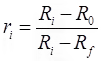where, Ri = Initial reading of dial gauge.
R= Reading of dial gauge at 0% consolidation.
Rf = Final reading of dial gauge after secondary consolidation.
2. Primary Consolidation Ratio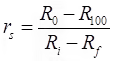where, R100 = Reading of dial gauge at 100% primary consolidation.
3. Secondary Consolidation Ratio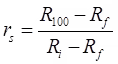ri+rp+rs = 1

Total Settlement

S = S+ Sp + Ss where, Si = Initial settlement
Sp = Primary settlement
S= Secondary settlement

1. Initial Settlement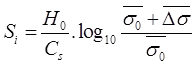For cohesionless soil.
where, Cs = 1.5(Cr0)
where, Cr = Static one resistance in kN/m2
H0 = Depth of soil sample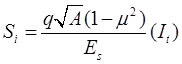For cohesive soil.
where, It = Shape factor or influence factor
A = Area.
2. Primary Settlement
(i)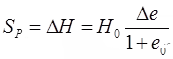(ii)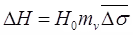(iii)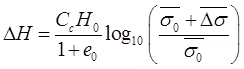(iv)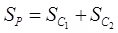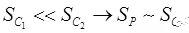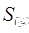= Settlement for over consolidated stage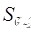= Settlement for normally consolidation stage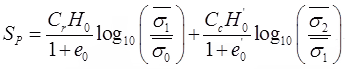3. Secondary Settlement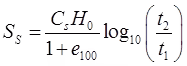where, H0∼H100
100 = Thickness of soil after 100% primary consolidation.
e100 = Void ratio after 100% primary consolidation.
t2 = Average time after t1 in which secondary consolidation is calculated

Permeability

1. Permeability of Soil
The permeability of a soil is a property which describes quantitatively, the ease with which water flows through that soil.
2. Darcy's Law
Darcy established that the flow occurring per unit time is directly proportional to the head causing flow and the area of cross-section of the soil sample but is inversely proportional to the length of the sample.
(i) Rate of flow (q)
qα(Δh/L)A → q = KiA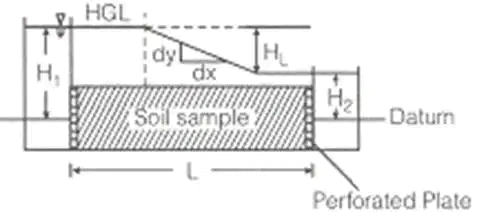Where, q = rate of flow in m3/sec.
K = Coefficient of permeability in m/s
A = Area of cross-section of sample
i = HL/L where, HL = Head loss = (H1 – H2)
i = tanθ(dy/dx)
(ii) Seepage velocity
Vs = V/n where, Vs = Seepage velocity (m/sec)
n = Porosity & V = discharge velocity (m/s)
(iii) Coefficiency of percolation
KP = K/n where, KP = coefficiency of percolation and n = Porosity.
K = QL/tHLA where, Q = Volume of water collected in time t in m3.
Constant Head Permeability test is useful for coarse grain soil and it is a laboratory method.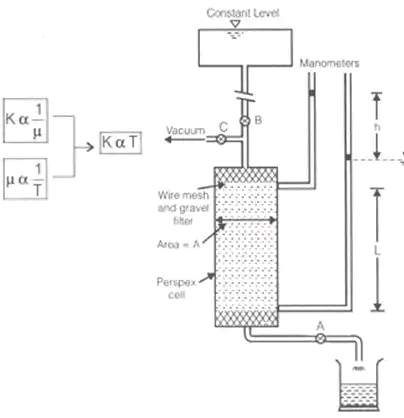K = 2.303aL/At(log10)(h/ h2)
a = Area of tube in m2
A = Area of sample in m2
t = time in 'sec'
L = length in 'm'
h1 = level of upstream edge at t = 0
h= level of upstream edge after 't'.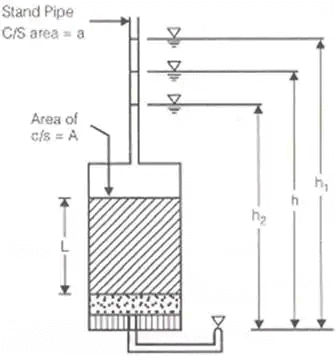5. Konzey-Karman Equation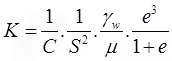Where, C = Shape coefficient, ∼5mm for spherical particle
S = Specific surface area = Area/Volume
6. For spherical particle.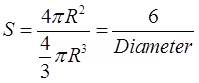R = Radius of spherical particle.
S = 6/√ab
When particles are not spherical and of variable size. If these particles passes through sieve of size 'a' and retain on sieve of size 'n'.
e = void ratio
μ = dynamic viscosity, in (N - s/m2)
γw = unit weight of water in kN/m3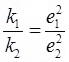7. Allen Hazen Equation
K=C.D210 Where, D10 = Effective size in cm. k is in cm/s C = 100 to 150
8. Lioudens Equation
log10KS2= a + b.n
Where, S = Specific surface area
n = Porosity.
a and b are constant.
Consolidation equation K = Cvmvγw
Where, C= Coefficient of consolidation in cm2/sec
mv = Coefficient of volume Compressibility in cm2/N
9. Capillary Permeability Test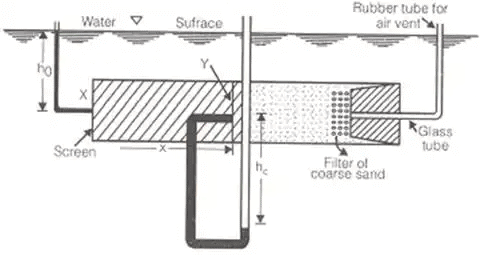i = h+ hc/x where, S = Degree of saturation
K = Coefficient of permeability of partially saturated soil.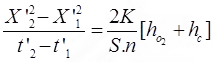where hc = remains constant (but not known as depends upon soil)
= head under first set of observation,
n = porosity, hc = capillary height
Another set of data gives,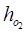= head under second set of observation
For S = 100%, K = maximum. Also, ku ∝ S.
10. Permeability of a stratified soil
(i) Average permeability of the soil in which flow is parallel to bedding plane,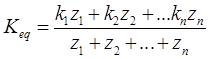keq∼kx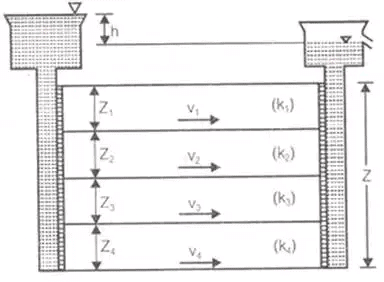(ii) Average permeability of soil in which flow is perpendicular to bedding plane.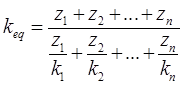keq∼kz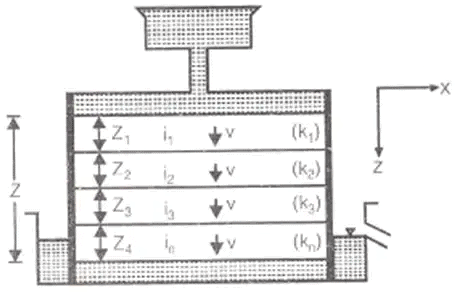(iii) For 2-D flow in x and z direction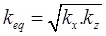(iv) For 3-D flow in x, y and z direction keq = (kx.ky.kz)1/3
Coefficient of absolute permeability (k0)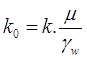Effective Stress, Capilarity, Seepage

1. Seepage Pressure and Seepage Force
Seepage pressure is exerted by the water on the soil due to friction drag. This drag force/seepage force always acts in the direction of flow.
The seepage pressure is given by
PS = hγω where, Ps = Seepage pressure
γω = 9.81 kN/m3
Here, h = head loss and z = length
(i) FS = hAγω where, Fs = Seepage force
(ii) fs = iγω where, fs = Seepage force per unit volume.
i = h/z where, I = Hydraulic gradient.
2. Quick Sand Condition
It is condition but not the type of sand in which the net effective vertical stress becomes zero, when seepage occurs vertically up through the sands/cohesionless soils.
Net effective vertical stress = 0
ic = (G - 1)/(1 + e) where, ic = Critical hydraulic gradient.
2.65 ≤ G ≤ 2.70 0.65 ≤ e ≤ 0.70
To Avoid Floating Condition
i < i and F.O.S = ic/i > 1

Laplace Equation of Two Dimensional Flow and Flow Net: Graphical Solution of Laplace Equation
(i)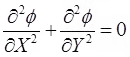where, ∅ = Potential function = kH
H = Total head and k = Coefficient of permeability
(ii)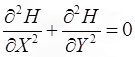… 2D Laplace equation for Homogeneous soil.
where, ∅ = kX H and ∅ = ky H for Isotropic soil, kx= ky

Seepage discharge (q)
q = kh.(Nf/Nd) where, h = hydraulic head or head difference between upstream and downstream level or head loss through the soil.

• Shape factor = Nf/Nd
• Nf = Nψ - 1
where, N= Total number of flow channels
Nψ = Total number of flow lines.
• Nd = N∅ - 1
where, Nd = Total number equipotential drops.
N∅ = Total number equipotential lines.
• Hydrostatic pressure = U = hwγw
where, U = Pore pressure hw = Pressure head
• Seepage Pressure
Ps = h'γw where, h' = h - (2h/Nd)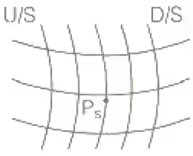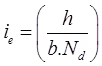where, size of exit flow field is b x b.
and ΔH = h/Nd is equipotential drop.

Phreatic Line

It is top flow line which follows the path of base parabola. It is a stream line. The pressure on this line is atmospheric (zero) and below this line pressure is hydrostatic.

1. Phreatic Line with Filter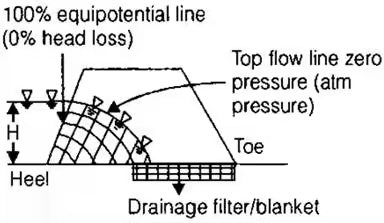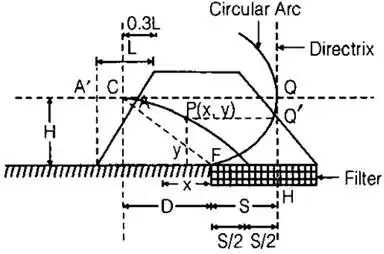Phreatic line (Top flow line).

Follows the path of base parabola
CF = Radius of circular arc =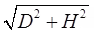C = Entry point of base parabola
F = Junction of permeable and impermeable surface
S = Distance between focus and directrix
= Focal length.
FH = S
(i) q = ks where, q = Discharge through unit length of dam.
(ii)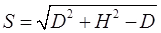(iii)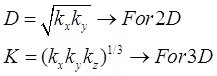2. Phreatic Line without Filter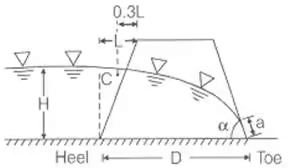(i) For ∝ < 30°
q = k a sin2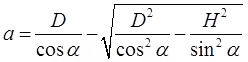(ii) For ∝ > 30°
q = k a sin ∝ tan ∝ and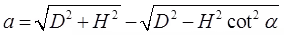The document Compressibility & Consolidation of Soils - Notes | Study Soil Mechanics - Civil Engineering (CE) is a part of the Civil Engineering (CE) Course Soil Mechanics.
All you need of Civil Engineering (CE) at this link: Civil Engineering (CE)

## Soil Mechanics

30 videos|50 docs|66 tests
 Use Code STAYHOME200 and get INR 200 additional OFF

## Soil Mechanics

30 videos|50 docs|66 tests

Track your progress, build streaks, highlight & save important lessons and more!

,

,

,

,

,

,

,

,

,

,

,

,

,

,

,

,

,

,

,

,

,

;